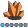SuzyG_
Calcite | Level 5

## Comparing variable order between 2 datasets

We use proc compare to validate dataset output--that both datasets have the same records, same number of variables, and all values match.  But is there a fast-n-easy way to compare the *order* of the variables?  If I have one dataset with columns left to right VAR1 VAR2 VAR3, and another dataset with columns left to right VAR2 VAR3 VAR1, proc compare only catches if there is a difference in the values or number of records.  I'm looking for a way to validate that the variables are in the order we expect, and just wondered if there was a SAS option or command or macro that will do this quickly?

Many thanks,

Suzy

3 REPLIES 3

## Re: Comparing variable order between 2 datasets

you can do:

data new;

retain VAR1 VAR2 VAR3;

set existing_data;run;

hope it helps!

## Re: Comparing variable order between 2 datasets

Hi Suzy,

The way i know is the use of proc contents, output dataset with variable name and order. Then comparing the two datasets. Hope it helps

data test;

d=2;

b001 =1;

b002 =2;

b003 =3;

b001z=1;

B001a=2;

CaSeSeNsItIvE2=9;

CASESENSITIVE3=9;

D=2;

casesensitive1=9;

CaSeSeNsItIvE1a=9;

d001z=1;

CASESENSITIVE1C=9;

D001a=2;

casesensitive1b=9;

A =1;

a002 =2;

a =3;

a001z=1;

A001a=2;

run;

data test2;

casesensitive1=9;

CaSeSeNsItIvE1a=9;

d001z=1;

CASESENSITIVE1C=9;

D001a=2;

casesensitive1b=9;

A =1;

a002 =2;

a =3;

a001z=1;

A001a=2;

d=2;

b001 =1;

b002 =2;

b003 =3;

b001z=1;

B001a=2;

CaSeSeNsItIvE2=9;

CASESENSITIVE3=9;

D=2;

run;

proc contents data=test out=ord(keep=name varnum) order=varnum;

run;

proc contents data=test2 out=ord2(keep=name varnum) order=varnum;

run;

proc compare base=ord compare=ord2 listall;

id name;

run;

Thanks,

Jag

Thanks,
JagPGStats
Opal | Level 21

## Re: Comparing variable order between 2 datasets

One way to get a list of out of order variables:

proc sql;

create table expect (var1 real, var2 real, var3 real);

create table other  (var2 real, var3 real, var1 real);

quit;

title "Variables out of order in dataset OTHER";

proc sql;

select *

from

(   select name, varnum "Wrong Order" from dictionary.columns

where libname = "WORK" and memname="OTHER" )

except

(   select name, varnum from dictionary.columns

where libname = "WORK" and memname="EXPECT" );

quit;

PG

PG
Discussion stats
• 3 replies
• 1728 views
• 1 like
• 4 in conversation